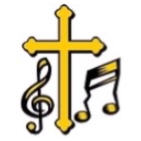Gospel Music by Ear by Reve' M. PeteLearn to Play Traditional Gospel Music by Ear!
 Home About the Course Begin the Course Dr. Reve' M. Pete Shop Links YouTube Contact
 Course Phases

#### Lesson Keywords

 Tweets by @albanysinternet

## Introduction to Chords

A chord consists of two or more tones played simultaneously. The tones used to play a chord are called chord tones. Tones of a major scale that are not used when a chord is played are called non-chord tones. Chord tones are identified using scale degrees.

The first degree of a major scale is called the root note. Its symbol is R. The root note is the tone around which a chord is built.

 C major Scale Degrees showing the Root Note
 Middle C = 1 = R D = 2 E = 3 F = 4 G = 5 A = 6 B = 7 C Octave = 8

Three tones played simultaneously are called a triad. Therefore a triad is a chord. Five triads will be studied in this course. They are the major triad, the minor triad, the augmented triad, the lowered fifth triad and the diminished triad. These triads are variations of the root note, third degree and fifth degree played simultaneously.

The major triad consists of the root note, third degree and fifth degree played simultaneously. The minor triad consists of the root note, third degree flat and fifth degree played simultaneously. The augmented triad consists of the root note, third degree and fifth degree sharp played simultaneously. The lowered fifth triad consists of the root note, third degree and fifth degree flat played simultaneously. The diminished triad consists of the root note, third degree flat and fifth degree flat played simultaneously.

Chords are played with the chord tones ( or pitches) in a specific order. This order is called a position. Triads played with the root note as the lowest pitch, third degree as the middle pitch and the fifth degree as the highest pitch in the chord are played in the root position. The order of chord tones in a triad may be rearranged. The rearrangement of chord tones is called an inversion. There are two inversions. They are the first inversion and the second inversion. The first inversion is also called the second position. The second inversion is also called the third position.

 C major triad (Scale Notes and Degrees)
 Middle C = 1 = R E = 3 G = 5

Accidentals are used to symbolize the alteration of a scale degree. However, the notation for altered degrees differs from the notation of altered scale notes. When notating an altered degree, the accidental is placed before the degree. The fifth degree sharp would be notated as #5. (When indicating an altered scale note, the accidental is placed after the note. G-sharp would be notated as G#.)

 C major triad Root Position
 Middle C = 1 = R E = 3 G = 5

 C minor triad Root Position
 Middle C = 1 = R Eb = b3 G = 5

 C augmented triad Root Positon
 Middle C = 1 = R E = 3 G# = #5

 C lowered fifth triad Root Positon
 Middle C = 1 = R E = 3 Gb = b5

 C diminished triad Root Positon
 Middle C = 1 = R Eb = b3 Gb = b5

The first inversion (or second position) of a triad is an arrangement of chord tones where the third degree is the lowest pitch, the fifth degree is the middle pitch and the eighth degree is the highest pitch. The eighth degree is the same note as the root note. However, the eighth degree is eight major scale notes (one octave) higher in pitch than the root note.

 C major triad First Inversion
 E = 3 G = 5 C Octave = 8

 C minor triad First Inversion
 Eb = b3 G = 5 C Octave = 8

 C augmented triad First Inversion
 E = 3 G# = #5 C Octave = 8

 C lowered fifth triad First Inversion
 E = 3 Gb = b5 C Octave = 8

 C diminished triad First Inversion
 Eb = b3 Gb = b5 C Octave = 8

The second inversion (or third position) of a triad is an arrangement of chord tones in which the fifth degree is the lowest pitch, the eighth degree is the middle pitch and the tenth degree is the highest pitch. The tenth degree is the same note as the third degree. However, the tenth degree is eight major scale notes (one octave) higher in pitch than the third degree.

 C major triad Second Inversion
 G = 5 C Octave = 8 E = 10

 C minor triad Second Inversion
 G = 5 C Octave = 8 Eb = b10

 C augmented triad Second Inversion
 G# = #5 C Octave = 8 E = 10

 C lowered fifth triad Second Inversion
 Gb = b5 C Octave = 8 E = 10

 C diminished triad Second Inversion
 Gb = b5 C Octave = 8 Eb = b10Web This Site
 The words "Gospel Music by Ear are a registered service mark of Reve' M. Pete Ministries, Inc. All rights reserved. Web site design by Albany's Internet Mall.